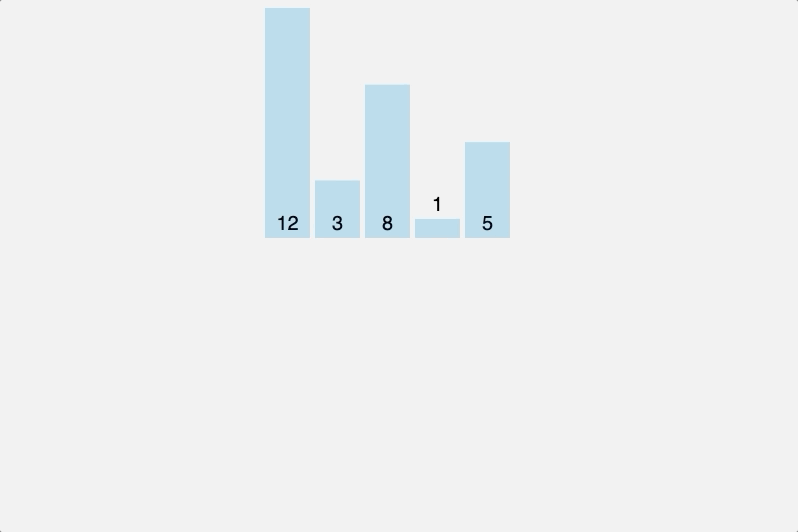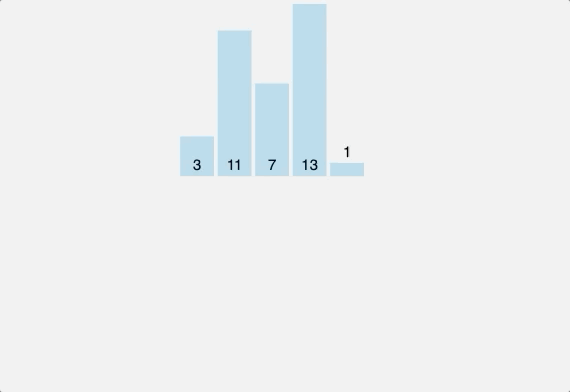# Sorting Algorithms: Insertion Sort

Insertion sort is one of many sorting algorithms. If you are new to sorting algorithms, I recommend you hop over to my first blog on sorting algorithms: “Sorting Algorithms: Bubble Sort”, where I go over some of the fundamental concepts of sorting algorithms, like swap.

What is Insertion Sort and How does it work?
In insertion sort, we start to sort our array by selecting and taking out one element. If there is a previous element in the array, we compare the two values. Based on the logic of our algorithm, we either have the two values switch places, or we insert the selected element back into the array before moving on to select the next element. As we continue iterating through the array, using the logic of our algorithm, we compare the next selected element to a previous element, and again we either have the two elements switch their respective indices or insert the selected element back into the array. Let’s say we want to sort from least to greatest, if there are more values in the array that are less than the selected value, we continue switching indices, until the selected element is inserted in the appropriate index. So if the selected element has a lower value than the previous element we switch their places in the array and continue this process of switching to the left, until the selected element gets inserted to the correct index. The higher value will then be next in line to be selected. We repeat this process going to the right until we have a sorted array.Visual of Insertion Sort Algorithm sorting an array of 12, 3, 8, 1, 5

As we sort, we begin to build a sorted section on the left side of our array. Now think that as we iterate, we are comparing a selected value to the previous value and then the previous value after that until the selected element can be inserted into our sorted section. As we continue iterating and sorting, what we are doing is gradually building out the sort, going from left to right. Compare this to Bubble sort and Selection sort and instead of finding one large or small element at a time and swapping them, the insertion algorithm takes each new element and places it where it should go within the sorted portion to the left.

This algorithm, I think is one of the easiest to understand. It’s best used in situations where we are taking in new data at the end of an array to be inserted.

Pseudo Code
• First we declare insertionSort as a function that takes an array as an argument.
• We select the second element in the array, create a variable called current to hold the value of the selected element
• Then we compare the selected element to the previous element to swap if necessary.
• Continue to the next element, and if it should need to be swapped with the previous value, continue swapping until it is inserted in the correct spot.
• Repeat this process until the array is sorted.

`function insertionSort(array){   for (let i = 1; i < array.length; i++){     let current = array[i];     for(let j = i - 1; j >= 0 && array[j] > current; j--){       array[j+1] = array[j]     }     array[j+1] = current  }  return array;}insertionSort([3,11,7,13,1])`

Let’s break this down. We start with our first loop beginning at index 1 because otherwise there would be no previous value to compare to the element in index 0. In the next line, the variable “current” is equal to the value of the element in index 1 of our array. In the example above, the value of array would be 11. In the nested loop that follows, we are declaring a variable j which will be equal to 1 minus the index of the current element. If j is used as an index, it will at first be the previous element in the array. As long as j is greater than 0 and congruently, the element at the index of j is greater than our current variable, we will swap the two values. However if one or both conditions mentioned are not true, then we will set the index of whatever j plus 1 equal to the current value. Let’s use the example above to demonstrate.

`let a = [3, 11, 7, 13, 1]insertionSort(a) //i=1   //current=11    //j=0, a[j]=3    //j >= 0 && a[j] > current ?       //=> is 0 >= 0 && 3 > 11 ?   //False i++   //a[j+1] = current //=> a = 11   //[3, 11, 7, 13, 1]//i=2  //current=7,     //j=1, a[j]=11    //j >= 0 && a[j] > current ?       //=> is 1 >= 0 && 11 > 7 ?       //True         //a[j+1] = a[j]         //=> a = a //=> a = 11 [3,7,11...]       //j--     //j=0, a[j]=3    //j >= 0 && a[j] > current ?       //=> is 0 >= 0 && 3 > 7 ?   //False i++   //a[j+1] = current //=> a = 7  //[3, 7, 11, 13, 1]//i=3... //Fastforward [3, 7, 11, 13, 1]//i=4  //current=1    //j=3, a[j]=13    //j >= 0 && a[j] > current ?       //=> is 3 >= 0 && 13 > 1 ?       //True         //a[j+1] = a[j]         //=> a = a //=> a = 13 [3,7,11,13,13]      //j--     //j=2, a[j]=11    //j >= 0 && a[j] > current ?        //=> is 2 >=0 && 11 > 1 ?       //True         //a[j+1] = a[j]        //=> a = a //=> a = 11 [3,7,11,11,13]      //j--    //j=1, a[j]=7    //j >= 0 && a[j] > current ?       //=> is 1 >=0 && 7 > 1 ?       //True         //a[j+1] = a[j]        //=> a = a //=> a = 7 [3,7,7,11,13]      //j--     //j=0, a[j]=3    //j >= 0 && a[j] > current ?       //=> is 0 >=0 && 3 > 1 ?       //True         //a[j+1] = a[j]        //=> a = a //=> a = 3 [3,3,7,11,13]      //j--       //Breaks because j is not >= 0   //j=-1   //a[j+1] = current //=> a = 1  //[1,3,7,11,13]`

I used this final example to showcase insertion sort in almost the best light. Insertion sort works best when we are inserting new data into the end of a nearly sorted or sorted array. To conclude, the final gif below will show the shorting process of the commented code above. I hope this explanation helps clarify the process of the Insertion Sort.Visual of Insertion Sort Algorithm sorting an array of 3, 11, 7, 13, 1

A Software Engineer with a background in Education Technology and Dance. Recent grad form FlatIron Bootcamp, and passion for the arts and working with databases

## More from Stephen Galvan

A Software Engineer with a background in Education Technology and Dance. Recent grad form FlatIron Bootcamp, and passion for the arts and working with databases